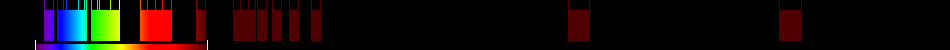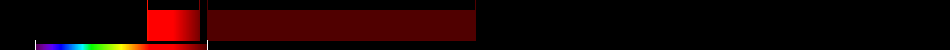# Information for Sensor and Index

## Formula

General Formula $\frac{{\mathrm{NIR}}}{{\mathrm{RED}}+\frac{{b}}{{a}}}$ $\frac{{\mathrm{18}}}{{\mathrm{12}}+\frac{{b}}{{a}}}$ Automatic

## Sensor

Name MERSI Medium Resolution Spectral Imager 20 402-12750 250-1000 0 Chinese FengYun-3 (FY-3A) China 2008-06-00 yes

## Index

Name Soil-adjusted vegetation index 2 SAVI2 $\frac{{\mathrm{NIR}}}{{\mathrm{RED}}+\frac{{b}}{{a}}}$ The actual gain (a) and intercept (b) values of the soil line rather than assuming them to be 1 and 0, respectively 640:760,780:1400 Original formula

## References